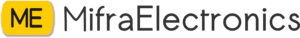Sale!

# 188 K Ohm resistors

20.00

## What is Resistor?

Resistor is defined as

A passive electrical component with two terminals that are used for either limiting or regulating the flow of electric current in electrical circuits.

The main purpose of resistor is to reduce the current flow and to lower the voltage in any particular portion of the circuit. It is made of copper wires which is coiled around a ceramic rod and the outer part of the resistor is coated with an insulating paint.

### What is the SI Unit of Resistor?

The SI unit of resistor is Ohm.

Availability: 50 in stock

SKU: MT0832 Category:

## What is Color Coding of Resistors?

Resistors may not display the value outside but resistor color pattern through their resistance can be calculated. PTH (plated-through-hole) resistors use a color-coding system (which really adds some flair to circuits), and SMD (surface-mount-device)resistors have their own value-marking system.

Following is a table with color code of resistors:

 Color Color code Black 0 Brown 1 Red 2 Orange 3 Yellow 4 Green 5 Blue 6 Violet 7 Grey 8 White 9

### What is Tolerance in Resistors?

Following is a table with tolerance of resistor:

 Colour Tolerance Brown ±1% Red ±2% Gold ±5% Silver ±10%

## Resistors in Series

Resistors are said to be in series when the current flowing through all the resistors is the same. These resistors are connected from head to tail in series. The overall resistance of the circuit is equal to the sum of individual resistance values.

### Resistors in Series Formula

 Rtotal = R1 + R2 + R3 +……+Rn

Where,

• Rtotal is the sum of all the individual resistances

## Resistors in Parallel

Resistors are said to be in parallel when the terminals of resistors are connected to the same two nodes. Resistors in parallel share the same voltage at their terminals.

### Resistors in Parallel Formula

 $$\frac{1}{R_{total}}=\frac{1}{R_{1}}+\frac{1}{R_{2}}+…..+\frac{1}{R_{n}}$$

Where,

• $$\frac{1}{R_{total}}$$ is the sum of all the individual resistances

## Applications of Resistor

Following are the applications of resistors:

• Wire wound resistors find application where balanced current control, high sensitivity, and accurate measurement are required like in shunt with ampere meter.
• Photo resistors find application in flame detectors, burglar alarm, in photographic devices, etc.
• Resistors are used for controlling temperature and voltmeter.
• Resistors are used in digital multi-meter, amplifiers, telecommunication, and oscillators.
• They are also used in modulators, demodulators, and transmitters.
• Linear Resistors
• Fixed resistors. Fixed resistors are resistors with a specific value. …
• Types of Fixed Resistors. Wire Wound Resistors. …
• Variable Resistors. …
• Types of Variable Resistors. …
• Thermistors. …
• Varistor Resistors. …
• Photo Resistor or LDR (Light Dependent Resistors) …
• Surface Mount Resistors.resistor is a passive two-terminal electrical component that implements electrical resistance as a circuit element. Resistors reduce current flow in electronic circuits, a property that is utilized to adjust signal levels, to divide voltagesbias active elements, and terminate transmission lines, among other uses. High-power resistors that can dissipate many watts of electrical power as heat may be used as part of motor controls, in power distribution systems, or as test loads for generators. Fixed resistors have resistances that only change slightly with temperature, time or operating voltage. Variable resistors can be used to adjust circuit elements (such as a volume control or a lamp dimmer), or as sensing devices for heat, light, humidity, force, or chemical activity.

Resistors are common elements of electrical networks and electronic circuits and are ubiquitous in electronic equipment. Practical resistors as discrete components can be composed of various compounds and forms. Resistors are also implemented within integrated circuits.

The electrical function of a resistor is specified by its resistance: common commercial resistors are manufactured over a range of more than nine orders of magnitude. The nominal value of the resistance falls within the manufacturing tolerance, indicated on the component.

## How resistors work

People who make electric or electronic circuits to do particular jobs often need to introduce precise amounts of resistance. They can do that by adding tiny components called resistors. A resistor is a little package of resistance: wire it into a circuit and you reduce the current by a precise amount. From the outside, all resistors look more or less the same. As you can see in the top photo on this page, and the one below, a resistor is a short, worm-like component with colored stripes on the side. It has two connections, one on either side, so you can hook it into a circuit.

What’s going on inside a resistor? If you break one open, and scratch off the outer coating of insulating paint, you might see an insulating ceramic rod running through the middle with copper wire wrapped around the outside. A resistor like this is described as wire-wound. The number of copper turns controls the resistance very precisely: the more copper turns, and the thinner the copper, the higher the resistance. In smaller-value resistors, designed for lower-power circuits, the copper winding is replaced by a spiral pattern of carbon. Resistors like this are much cheaper to make and are called carbon-film. Generally, wire-wound resistors are more precise and more stable at higher operating temperatures.

• keep in touch with our social media links as mentioned below
Mifratech Note : Find the best solution for electronics components and technical projects ideas
mifratech instagram : https://www.instagram.com/mifratech/